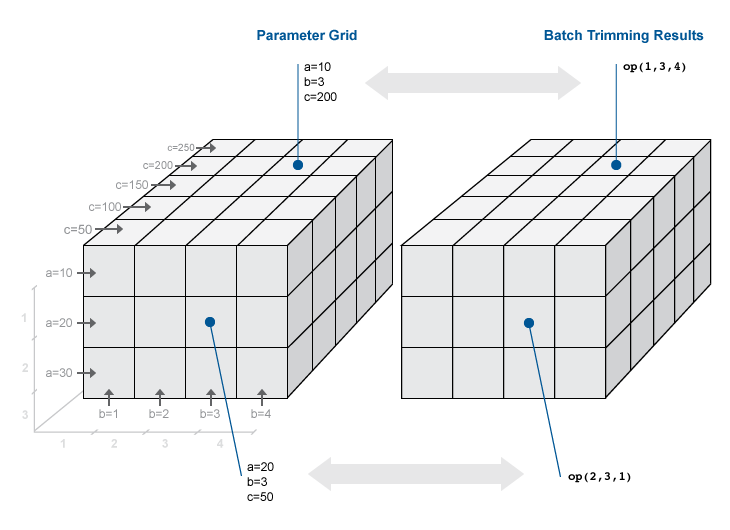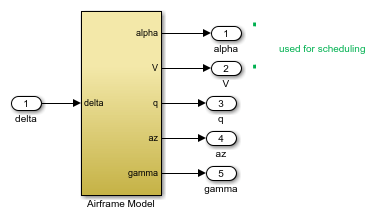Documentation

This is machine translation

Mouseover text to see original. Click the button below to return to the English version of the page.

Batch Compute Steady-State Operating Points for Parameter Variation

Block parameters configure a Simulink® model in several ways. For example, you can use block parameters to specify various coefficients or controller sample times. You can also use a discrete parameter, like the control input to a Multiport Switch block, to control the data path within a model. Varying the value of a parameter helps you understand its impact on the model behavior. Also, you can vary the parameters of a plant model in a control system to study the robustness of the controller to plant variations.

When trimming a model using `findop`, you can specify a set of parameter values for which to trim the model. The full set of values is called a parameter grid or parameter samples. `findop` computes an operating point for each value combination in the parameter grid. You can vary multiple parameters, thus extending the parameter grid dimension.

Which Parameters Can Be Sampled?

You can vary any model parameter with a value given by a variable in the model workspace, the MATLAB® workspace, or a data dictionary. In cases where the varying parameters are all tunable (Simulink), `findop` requires only one model compilation to find operating points for varying parameter values. This efficiency is especially advantageous for models that are expensive to compile repeatedly.

Vary Single Parameter

To vary the value of a single parameter for batch trimming with `findop`, specify the parameter grid as a structure having two fields. The `Name` field contains the name of the workspace variable that specifies the parameter. The `Value` field contains a vector of values for that parameter to take during trimming.

For example, the `Watertank` model has three parameters defined as MATLAB workspace variables, `a`, `b`, and `A`. The following commands specify a parameter grid for the single parameter for `A`.

```param.Name = 'A'; param.Value = Avals;```

Here, `Avals` is an array specifying the sample values for `A`.

The following table lists some common ways of specifying parameter samples.

Parameter Sample-Space TypeHow to Specify the Parameter Samples
Linearly varying`param.Value = linspace(A_min,A_max,num_samples)`
Logarithmically varying`param.Value = logspace(A_min,A_max,num_samples)`
Random`param.Value = rand(1,num_samples)`
Custom`param.Value = custom_vector`

If the variable used by the model is not a scalar variable, specify the parameter name as an expression that resolves to a numeric scalar value. For example, suppose that `Kpid` is a vector of PID gains. The first entry in that vector, `Kpid`, is used as a gain value in a block in your model. Use the following commands to vary that gain using the values given in a vector `Kpvals`:

```param.Name = 'Kpid(1)'; param.Value = Kpvals;```

After you create the structure `param`, pass it to `findop` as the `param` input argument.

Multidimension Parameter Grids

When you vary more than one parameter at a time, you generate parameter grids of higher dimension. For example, varying two parameters yields a parameter matrix, and varying three parameters yields a 3-D parameter grid. Consider the following parameter grid used for batch trimming:Here, you vary the values of three parameters, a, b, and c. The samples form a 3-by-4-by-5 grid. `op` is an array with same dimensions that contains corresponding trimmed operating point objects.

Vary Multiple Parameters

To vary the value of multiple parameters for batch trimming with `findop`, specify parameter samples as a structure array. The structure has an entry for each parameter whose value you vary. The structure for each parameter is the same as described in Vary Single Parameter. You can specify the `Value` field for a parameter as an array of any dimension. However, the size of the `Value` field must match for all parameters. Corresponding array entries for all the parameters, also referred to as a parameter grid points, must map to a specified parameter combination. When the software trims the model, it computes an operating point for each grid point.

Specify Full Grid

Suppose that your model has two parameters whose values you want to vary, a and b:

`$\begin{array}{l}a=\left\{a1,a2\right\}\\ b=\left\{b1,b2\right\}\end{array}$`

You want to trim the model for every combination of a and b, also referred to as a full grid:

`$\left\{\begin{array}{cc}\left({a}_{1},{b}_{1}\right),& \left({a}_{1},{b}_{2}\right)\\ \left({a}_{2},{b}_{1}\right),& \left({a}_{2},{b}_{2}\right)\end{array}\right\}$`

Create a rectangular parameter grid using `ndgrid`.

```a1 = 1; a2 = 2; a = [a1 a2]; b1 = 3; b2 = 4; b = [b1 b2]; [A,B] = ndgrid(a,b) ```
```>> A A = 1 1 2 2 >> B B = 3 4 3 4```

Create the structure array, `params`, that specifies the parameter grid.

```params(1).Name = 'a'; params(1).Value = A; params(2).Name = 'b'; params(2).Value = B; ```

In general, to specify a full grid for N parameters, use `ndgrid` to obtain N grid arrays.

`[P1,...,PN] = ndgrid(p1,...,pN);`

Here, `p1`,...,`pN` are the parameter sample vectors.

Create a 1 x N structure array.

```params(1).Name = 'p1'; params(1).Value = P1; ... params(N).Name = 'pN'; params(N).Value = PN; ```

Specify Subset of Full Grid

If your model is complex or you vary the value of many parameters, trimming the model for the full grid can become expensive. In this case, you can specify a subset of the full grid using a table-like approach. Using the example in Specify Full Grid, suppose that you want to trim the model for the following combinations of a and b:

`$\left\{\left({a}_{1},{b}_{1}\right),\left({a}_{1},{b}_{2}\right)\right\}$`

Create the structure array, `params`, that specifies this parameter grid.

```A = [a1 a1]; params(1).Name = 'a'; params(1).Value = A; B = [b1 b2]; params(2).Name = 'b'; params(2).Value = B; ```

Batch Trim Model for Parameter Variations

This example shows how to obtain multiple operating points for a model by varying parameter values. You can study the controller robustness to plant variations by batch linearizing the model using the trimmed operating points.

```sys = 'watertank'; open_system(sys) ```Vary parameters `A` and `b` within 10% of their nominal values. Specify three values for `A` and four values for `b`, creating a 3-by-4 value grid for each parameter.

```[A_grid,b_grid] = ndgrid(linspace(0.9*A,1.1*A,3),... linspace(0.9*b,1.1*b,4)); ```

Create a parameter structure array, specifying the name and grid points for each parameter.

```params(1).Name = 'A'; params(1).Value = A_grid; params(2).Name = 'b'; params(2).Value = b_grid; ```

Create a default operating point specification for the model, which specifies that both model states are unknown and must be at steady state in the trimmed operating point.

```opspec = operspec(sys) ```
``` Operating point specification for the Model watertank. (Time-Varying Components Evaluated at time t=0) States: ---------- (1.) watertank/PID Controller/Integrator/Continuous/Integrator spec: dx = 0, initial guess: 0 (2.) watertank/Water-Tank System/H spec: dx = 0, initial guess: 1 Inputs: None ---------- Outputs: None ---------- ```

By default, `findop` displays an operating point search report in the Command Window for each trimming operation. To suppress the report display, create a trimming option set and turn off the operating point search report display.

```opt = findopOptions('DisplayReport','off'); ```

Trim the model using the specified operating point specification, parameter grid, and option set.

```[op,opreport] = findop(sys,opspec,params,opt); ```

`findop` trims the model for each parameter combination. The software uses only one model compilation. `op` is a 3-by-4 array of operating point objects that correspond to the specified parameter grid points.

View the operating point in the first row and first column of `op`.

```op(1,1) ```
``` Operating point for the Model watertank. (Time-Varying Components Evaluated at time t=0) States: ---------- (1.) watertank/PID Controller/Integrator/Continuous/Integrator x: 1.41 (2.) watertank/Water-Tank System/H x: 10 Inputs: None ---------- ```

Batch Trim Model at Known States Derived from Parameter Values

This example shows how to batch trim a model when the specified parameter variations affect the known states for trimming.

In the Batch Trim Model for Parameter Variations example, the model is trimmed to meet a single operating point specification that contains unknown states. In other cases, the model states are known for trimming, but depend on the values of the varying parameters. In this case, you cannot batch trim the model using a single operating point specification. You must create a separate specification for each parameter value grid point.

```sys = 'scdairframeTRIM'; open_system(sys) ```In this model, the aerodynamic forces and moments depend on the speed,, and incidence,.

Vary theandparameters, and create a 6-by-4 parameter grid.

```nA = 6; % number of alpha values nV = 4; % number of V values alphaRange = linspace(-20,20,nA)*pi/180; vRange = linspace(700,1400,nV); [alphaGrid,vGrid] = ndgrid(alphaRange,vRange); ```

Since some known state values for trimming depend on the values ofand, you must create a separate operating point specification object for each parameter combination.

```for i = 1:nA for j = 1:nV % Set parameter values in model. alpha_ini = alphaGrid(i,j); v_ini = vGrid(i,j); % Create default specifications based on the specified parameters. opspec(i,j) = operspec(sys); % Specify which states are known and which states are at steady state. opspec(i,j).States(1).Known = [1;1]; opspec(i,j).States(1).SteadyState = [0;0]; opspec(i,j).States(3).Known = [1;1]; opspec(i,j).States(3).SteadyState = [0;1]; opspec(i,j).States(2).Known = 1; opspec(i,j).States(2).SteadyState = 0; opspec(i,j).States(4).Known = 0; opspec(i,j).States(4).SteadyState = 1; end end ```

Create a parameter structure for batch trimming. Specify a name and value grid for each parameter.

```params(1).Name = 'alpha_ini'; params(1).Value = alphaGrid; params(2).Name = 'v_ini'; params(2).Value = vGrid; ```

Trim the model using the specified parameter grid and operating point specifications. When you specify an array of operating point specifications and varying parameter values, the dimensions of the specification array must match the parameter grid dimensions.

```opt = findopOptions('DisplayReport','off'); op = findop(sys,opspec,params,opt); ```

`findop` trims the model for each parameter combination. `op` is a 6-by-4 array of operating point objects that correspond to the specified parameter grid points.